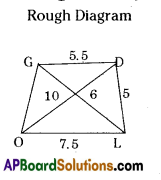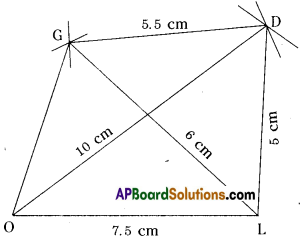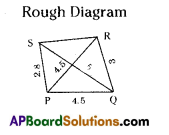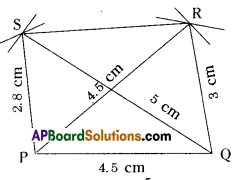AP State Syllabus AP Board 8th Class Maths Solutions Chapter 3 Construction of Quadrilaterals Ex 3.3 Textbook Questions and Answers.

## AP State Syllabus 8th Class Maths Solutions 3rd Lesson Construction of Quadrilaterals Exercise 3.3Construct the quadrilateral with the measurements given below :

Question a).
Quadrilateral GOLD: OL = 7.5 cm, GL = 6 cm, LD = 5 cm, DG = 5.5 cm and OD = 10 cm.
(Ex 3.3, Page No. 72)
Solution:
In a quadrilateral GOLD, Rough Diagram
OL = 7.5 cm, GL = 6 cm
LD = 5 cm, DG = 5.5 cm, OD = 10 cmConstruction Steps:

1. Draw a line segment $$\overline{OL}$$ equal to radius 7.5 cm.
2. With the centres O, L draw arcs with radius 10 cm and 5 cm respectively. These two arcs meet at point ‘D’.
3. With the centres L, D draw arcs equal to 6 cm and 5.5 cm respectively. These two arcs meet at point ‘G’.
4. Join O, G and L, G. Also join O, D and L, D and G, D.
5. ∴ The required quadrilateral GOLD is formed.Question b).
Quadrilateral PQRS: PQ = 4.2 cm, QR = 3 cm, PS = 2.8 cm, PR = 4.5 cm and QS = 5 cm.
Solution:1. Draw a line segment $$\overline{P Q}$$ with radius 4.2 cm.